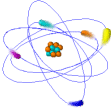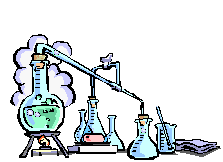# Chapter 2 Worksheet BI – Chemistry

 Chemistry WorksheetSection  2-1    Composition of Matter

1. Define matter.

2. Define mass.

3. Explain the difference between mass & weight.

4. Why do biologists study chemistry?

5. Define element.

6. Name the 4 elements that make up 90% of the mass of living things. Give the symbol for each of these elements.

7. Explain why some elements such as sodium have odd symbols.

8. Sketch a block from the periodic table and label the atomic number, atomic mass, & symbol for the element.

9. Define atom and tell whether they can be seen.

10. What is the center of an atom called & what 2 subatomic particles are found there?

11. How does the charge of a proton differ from the charge of a neutron?

12. Where is most of the mass of an atom concentrated?

13. How is the atomic number of an element determined?

14. What is the charge on an electron?

15. Explain why the overall or net charge on an atom is zero.

16. Where are electrons found in an atom & describe their movement?

17. In which energy levels do the electrons have more energy?

18. How many electrons can these energy levels hold   — a. first?        b. second?

19. Define compound and write a formula for water, carbon dioxide, & sodium chloride (table salt).

20. Do compounds have the same chemical properties as the elements that compose them?

21. When would an atom be chemically stable (not react)?

22. What occurs in a chemical reaction?

23. What is a covalent bond?

24. Define molecule.

25. Give an example of a gas that exists as a molecule.

26. Define ionic bond.

27. What is an ion?

28. Name a compound formed from — a. covalent bonding?            b. ionic bonding?

29. If electrons are shared, a(n) ______________ compound forms.

30. If electrons are transferred, a(n) _____________ compound forms.

31. Forming ionic or covalent bonds helps make atoms more ________________.

Section 2-2    Energy32. All living things require _____________ to do work.

33. Energy can’t be created or _____________ in a chemical reaction, but it can be _____________ from one form into another.

34. Name 4 forms of energy important to living things.

35. What is free energy?

36. Give an example of energy changing form in an organism.

37. Atoms & molecules are in constant _______________.

38. Name the 3 main states of matter.

39. Explain how the shape and volume of a solid, liquid, and gas differ.

40. Organisms undergo thousands of ____________ as part of their life processes.

41. Where are the reactants and products in a chemical equation?

42. What does a two-direction arrow mean in a chemical equation?

43. _______________ are broken down in chemical reactions in your body to release ___________ and produce _______________ and ______________.

44. What is the difference between an endergonic & exergonic reaction?

45. What is activation energy?

46. What effect does a catalyst have on activation energy?

47. What are biological catalysts called?

48. Redox is the abbreviation for what type of reaction?

49. Redox reactions involve the transfer of energy and _________ between atoms.

50. What happens during oxidation?

51. What happens during reduction?

52. Give an example of oxidation.

53. Give an example of reduction.

Section 2-3        Solutions

54. Many of the chemical reactions in organisms take place in __________.

55. What is a solution?

56. Give an example of a complex solution in your body.

57. Name & describe the 2 parts of a solution.

58. What is meant by concentration of the solution?

59. How do you get a saturated solution?

60. What are aqueous solutions?

61. Explain dissociation of water molecules.

62. Name and give the charge for the 2 ions formed whenever water dissociates.

63. Write the final equation for the dissociation of water.

64. What is the hydronium ion?

65. How are acidity and alkalinity measured?

66. When would a solution be neutral?    Give an example of a neutral solution.

67. When would solutions be considered as acidic?

68. Acids have what taste?

69. Acids form what ion in water?

70. Give an example of an acid in your stomach.

71. When would solutions be considered as a base?

72. What adjective refers to basic solutions?

73. Give an example of a base.

74. What ion forms whenever a base is dissolved in water?

75. How does a base taste and feel?

77. What is the pH scale used for?

78. What is the range for the pH scale?

79. At what pH would you find each of these solutions on a pH scale:    a. acids?    b. Bases?    c. neutral?

80. How many times stronger is a pH of 3 than a pH of 5?

81. A change of one pH unit reflects a __________ change.

82. Why is controlling the pH range important to organisms?

83. How do organisms control their pH levels?

84. What is a buffer?

85. Give an example of a human body fluid that is:    a. acidic?    b. alkaline?BACK